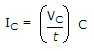# Electronics - Basic Op-Amp Circuits

Exercise : Basic Op-Amp Circuits - General Questions
1.
The center frequency of a band-pass filter is always equal to the
bandwidth
–3 dB frequency
bandwidth divided by Q
geometric average of the critical frequencies
Explanation:
No answer description is available. Let's discuss.

2.
The formulashows that for a given capacitor, if the voltage changes at a constant rate with respect to time, the current will
increase
decrease
be constant
decrease logarithmically
Explanation:
No answer description is available. Let's discuss.

3.
A zero-level detector is a
comparator with a sine-wave output
comparator with a trip point referenced to zero
peak detector
limiter
Explanation:
No answer description is available. Let's discuss.

4.
A digital-to-analog converter is an application of the
voltage-to-current converter
noninverting amplifier